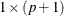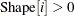## how to input shape parameter in randdirichlet

my data has 4 categories, and each category has count of 50, 100, 150, 200 (percentages as 10%, 20%, 30% and 40% of total data).

I want to simulate and sampling with randdirichlet function for these 4 percentages, but with the code below, I only got 3 percentages and have to calculate the 4th one myself. Maybe I did not specify the shape parameters correctly. Please advise.

/* want to simulate 4 percentages with randdirichlet*/
proc iml;
call randseed(1);
n = 1000;
Shape = {50, 100, 150, 200};	/* counts of each category */
x = RandDirichlet(n,Shape);  	/* x is 1000 x 3 matrix*/
samplemean=mean(x);  		/* check mean */
print samplemean;

varnames='percentage1':'percentage3';
create MyData from x[colname=varnames];
append from x;
close MyData;       /* only first 3 percentages columns */
quit;

/* to get the 4th percentage */
data mydata2;
set mydata;
percentage4=1-(percentage1+percentage2+percentage3);
run;

according to sas user's guide

Shape is avector of shape parameters for the distribution,and in the example, the shape has 3 parameters for a two-dimensional Dirichlet distribution

call randseed(1);
n = 1000;
Shape = {2, 1, 1};
x = RandDirichlet(n,Shape);

## Re: how to input shape parameter in randdirichlet

Because you mention counts and percentages, I think you are looking for the multinomial distribution, not the Dirichlet distribution, The multinomial distribution, which you can simulate by using the RANDMULTINOMIAL function in SAS/IML, generates random frequencies for k categories where the probabilities of the categories in the population are known. For example,

proc iml;
call randseed(1);
n = 1000;
counts = {50, 100, 150, 200};	/* expected count for each category */
total = sum(counts);
prob = counts / total;
x = RandMultinomial(n, total, prob); /* x is 1000 x 4 matrix of counts */
print (x[1:5,]);

The Dirichlet distribution is a multivariate generalization of the beta distribution, so I do not immediately see how it is related to counts.

From The DO Loop